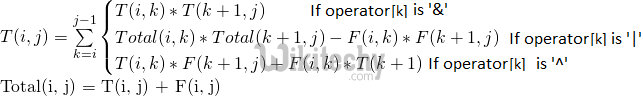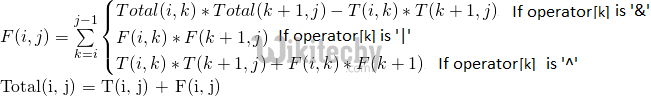# Boolean Parenthesization Problem

Boolean Parenthesization Problem - Dynamic Programming Count the number of ways we can parenthesize the expression so that the value of expression

Given a boolean expression with following symbols.

```Symbols
'T' ---> true
'F' ---> false```

And following operators filled between symbols

```Operators
&   ---> boolean AND
|   ---> boolean OR
^   ---> boolean XOR```

Count the number of ways we can parenthesize the expression so that the value of expression evaluates to true.

Let the input be in form of two arrays one contains the symbols (T and F) in order and other contains operators (&, | and ^}

Examples:

```Input: symbol[]    = {T, F, T}
operator[]  = {^, &}
Output: 2
The given expression is "T ^ F & T", it evaluates true
in two ways "((T ^ F) & T)" and "(T ^ (F & T))"

Input: symbol[]    = {T, F, F}
operator[]  = {^, |}
Output: 2
The given expression is "T ^ F | F", it evaluates true
in two ways "( (T ^ F) | F )" and "( T ^ (F | F) )".

Input: symbol[]    = {T, T, F, T}
operator[]  = {|, &, ^}
Output: 4
The given expression is "T | T & F ^ T", it evaluates true
in 4 ways ((T|T)&(F^T)), (T|(T&(F^T))), (((T|T)&F)^T)
and (T|((T&F)^T)).
```

Solution:
Let T(i, j) represents the number of ways to parenthesize the symbols between i and j (both inclusive) such that the subexpression between i and j evaluates to true.Let F(i, j) represents the number of ways to parenthesize the symbols between i and j (both inclusive) such that the subexpression between i and j evaluates to false.Base Cases:

```T(i, i) = 1 if symbol[i] = 'T'
T(i, i) = 0 if symbol[i] = 'F'

F(i, i) = 1 if symbol[i] = 'F'
F(i, i) = 0 if symbol[i] = 'T'```

If we draw recursion tree of above recursive solution, we can observe that it many overlapping subproblems. Like other dynamic programming problems, it can be solved by filling a table in bottom up manner. Following is C++ implementation of dynamic programming solution.

C++
``````#include<iostream>
#include<cstring>
using namespace std;

// Returns count of all possible parenthesizations that lead to
// result true for a boolean expression with symbols like true
// and false and operators like &, | and ^ filled between symbols
int countParenth(char symb[], char oper[], int n)
{
int F[n][n], T[n][n];

// Fill diaginal entries first
// All diagonal entries in T[i][i] are 1 if symbol[i]
// is T (true).  Similarly, all F[i][i] entries are 1 if
// symbol[i] is F (False)
for (int i = 0; i < n; i++)
{
F[i][i] = (symb[i] == 'F')? 1: 0;
T[i][i] = (symb[i] == 'T')? 1: 0;
}

// Now fill T[i][i+1], T[i][i+2], T[i][i+3]... in order
// And F[i][i+1], F[i][i+2], F[i][i+3]... in order
for (int gap=1; gap<n; ++gap)
{
for (int i=0, j=gap; j<n; ++i, ++j)
{
T[i][j] = F[i][j] = 0;
for (int g=0; g<gap; g++)
{
// Find place of parenthesization using current value
// of gap
int k = i + g;

// Store Total[i][k] and Total[k+1][j]
int tik = T[i][k] + F[i][k];
int tkj = T[k+1][j] + F[k+1][j];

// Follow the recursive formulas according to the current
// operator
if (oper[k] == '&')
{
T[i][j] += T[i][k]*T[k+1][j];
F[i][j] += (tik*tkj - T[i][k]*T[k+1][j]);
}
if (oper[k] == '|')
{
F[i][j] += F[i][k]*F[k+1][j];
T[i][j] += (tik*tkj - F[i][k]*F[k+1][j]);
}
if (oper[k] == '^')
{
T[i][j] += F[i][k]*T[k+1][j] + T[i][k]*F[k+1][j];
F[i][j] += T[i][k]*T[k+1][j] + F[i][k]*F[k+1][j];
}
}
}
}
return T[n-1];
}

// Driver program to test above function
int main()
{
char symbols[] = "TTFT";
char operators[] = "|&^";
int n = strlen(symbols);

// There are 4 ways
// ((T|T)&(F^T)), (T|(T&(F^T))), (((T|T)&F)^T) and (T|((T&F)^T))
cout << countParenth(symbols, operators, n);
return 0;
}``````

Output :

`4`

Time Complexity: O(n3)
Auxiliary Space: O(n2)